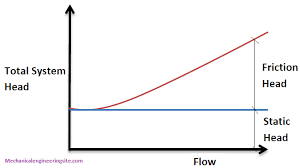## How to Calculate and Solve for Rated Static Head | Design of Gating SystemThe image above represents rated static head.

To compute for rated static head, three essential parameters are needed and these parameters are Height of the Sprue from its Top to the Point of Metal Entry into the Mould Cavity (H), Height of the Casting System from its top (P) and Height of the Casting (C).

The formula for calculating rated static head:

Hs = H – P2 / 2C

Where:

H = Height of the Sprue from its Top to the Point of Metal Entry into the Mould Cavity
P = Height of the Casting from its Top
C = Height of the Casting

Let’s solve an example;
Find the rated static head when the height of the sprue from its top to the point of metal entry into the mould cavity is 9,the height of the casting from its top is 5 and the height of the casting is 11.

This implies that;

H = Height of the Sprue from its Top to the Point of Metal Entry into the Mould Cavity = 9
P = Height of the Casting from its Top = 5
C = Height of the Casting = 11

Hs = H – P2 / 2C
Hs = 9 – 52 / 2 x 11
Hs = 9 – 25 / 22
Hs = 9 – 1.136
Hs = 7.86

Therefore, the rated static head is 7.86 m.

Calculating the Height of the Sprue from its Top to the Point of Metal Entry into the Mould Cavity when the Rated Static Head, Height of the Casting System from its Top and the Height of the Casting is Given.

H = HsP2 / 2C

Where;

H = Height of the Sprue from its Top to the Point of Metal Entry into the Mould Cavity
P = Height of the Casting from its Top
C = Height of the Casting

Let’s solve an example;
Find the height of the sprue when the rated static head is 25, the height of the casting system from its top is 8 and the height of the casting is 4.

This implies that;

Hs = Rated Static Head = 25
P = Height of the Casting from its Top = 8
C = Height of the Casting = 4

H = HsP2 / 2C
H = 25 + 82 / 2 x 4
H = 25 + 64 / 8
H = 25 + 8
H = 33

Therefore, the height of the casting system from its top is 33.